Curvature Tensors

Notation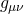is the metric,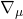is the covariant derivative, andis the partial derivative with respect to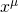. Square brackets surrounding indices denote antisymmetrization, and round brackets denote symmetrization. For example,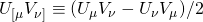and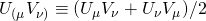.

Christoffel symbols

(1)Riemann tensor

The Riemann tensor or the Riemann-Christoffel curvature tensor is a four-index tensor describing the curvature of Riemannian manifolds.

(2)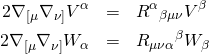Riemann, Ricci, curvature scalar, Einstein tensor, and Weyl tensor

(3)Weyl curvature tensor represents the traceless component of the Riemann curvature tensor.

(The definition of the Weyl tensor depends on the number of spacetime dimensions n. Here it is assumed that n=4.)

Symmetries

For Riemann, the three symmetries of the curvature tensor are:

\begin{array}{rcl}
R_{bacd} & = & -R_{abcd} \\
R_{abdc} & = & -R_{abcd} \\
R_{cdab} & = & R_{abcd} \\
R_{a[bcd]} & = & 0
\end{array}
The last symmetry, discovered by Ricci is called the first Bianchi identity or algebraic Bianchi identity. Given any tensor which satisfies these symmetries, one can completely describe a Riemannian manifold with the indicated curvature tensor at any point. The Ricci and Einstein tensors are symmetric.

Bianchi identities

\begin{array}{rcl}
\nabla_{[a} R_{bc]de} & = & 0 \\
\nabla_a G^{ab} & = & 0
\end{array}